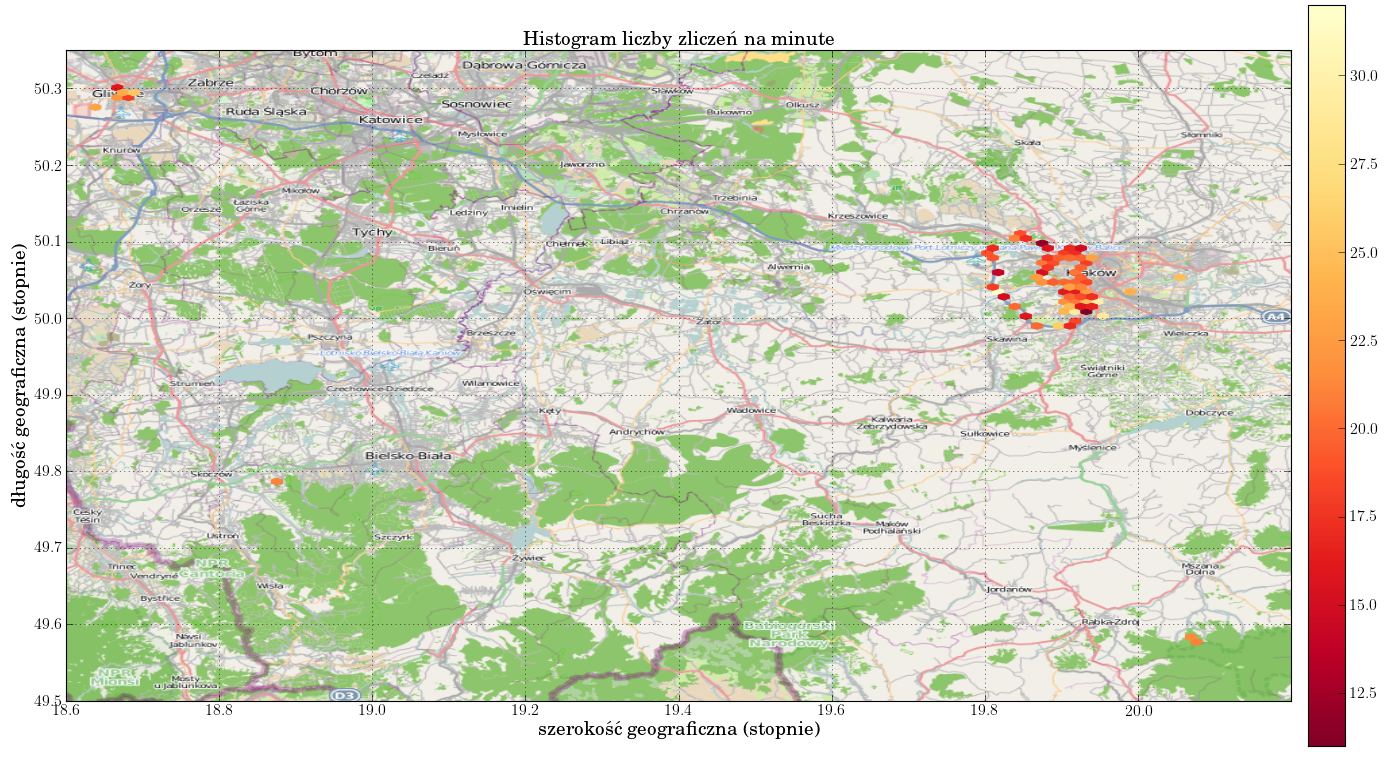# Openstreetmap maps in Python

I figured out a simple way to present data on a map in Python.

``````from urllib2 import urlopen
from pylab import imshow, imread, show

lon = [49.5,50.35]
lat = [18.6,20.2]
scale = 700000

# open an openstreetmap export png file via http
url = urlopen('http://parent.tile.openstreetmap.org/cgi-bin/export?'
'bbox={lat1:.2f},{lon1:.2f},{lat2:.2f},{lon2:.2f}&'
'scale={scale:d}&format=png'.format(lat1=lat,
lat2=lat,
lon1=lon,
lon2=lon,
scale=scale))

# plot the map

# plot other data here

show()
``````

Openstreetmap server often experiences heavy loads (everyone wants access to these tiles, including me). A little more code to handle denials:

``````from urllib2 import urlopen
from pylab import imshow, imread, show

lon = [49.5,50.35]
lat = [18.6,20.2]
scale = 700000

tries = 0
url = None
while tries < 60:
tries += 1
print 'Try {}...'.format(tries)
try:
url = urlopen('http://parent.tile.openstreetmap.org/cgi-bin/export?'
'bbox={lat1:.2f},{lon1:.2f},{lat2:.2f},{lon2:.2f}&'
'scale={scale:d}&format=png'.format(lat1=lat,
lat2=lat,
lon1=lon,
lon2=lon,
scale=scale))
except HTTPError:
sleep(5)
continue
else:
break

if url is None:
else:
Combined with matplotlib's `hexbin` one can create nice density plots.By the way: `hexbin` has an amazing parameter `C` (lacking in every other density plot function I've encountered). According to documentation: